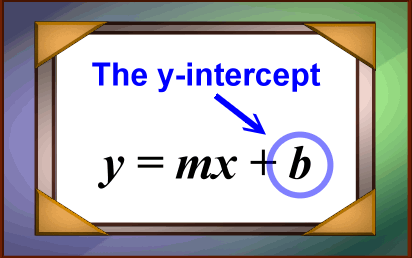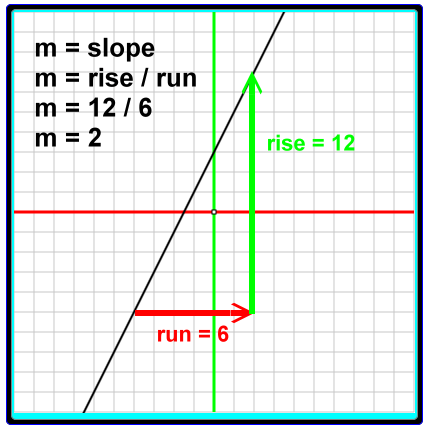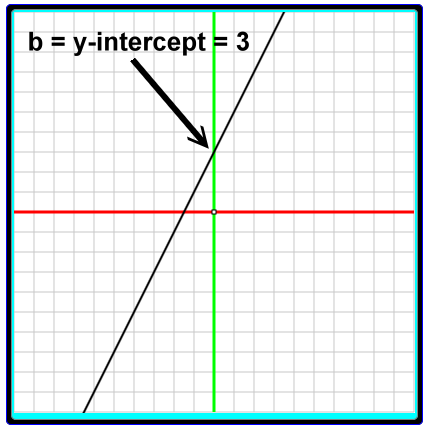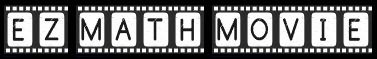# Linear Functions and Equations, Slope-Intercept Form

### Linear function interactive app (explanation below):

Here we have an application that let's you change the slope and y-intercept for a line on the (x, y) plane. You change these values by clicking on the '+' and '-' buttons. After each click the graph will be redrawn and the equation for the line will be redisplayed using the new values.

This is an EZ Math Movie. Click the 'Show system' checkbox to expose the EZ Math Movie language. You do not have to understand EZ Math Movie to use this application.

0ptions

Go on input

Bounds:
Sorry, apparently this browser does not support HTML 5 canvas tag graphics.
Printer

Edit: Runtime          View: Starter Suggestion

To runtime:

Storage:

Editor width: Normal Wide

### y = mx + b

The formula y = mx + b is said to be a linear function. That means the graph of this function will be a straight line on the (x, y) plane.

One could express this as a formal function definition with notation such as:

f(x) = mx + b

Since we will be graphing (x, y) points, though, we will do our thinking with the 'y = mx + b' form for a while.

When the function for a line is expressed this way, we call it the 'slope-intercept form'.

### Where is the slope?

The slope of the line is the variable m.The slope describes the slant of the line.

### Where is the intercept?

By 'intercept' we mean 'y-intercept'. The y-intercept is held by the variable b.The y-intercept is the point where the line crosses the y-axis.

### If you know the slope for the line....### ....and you know the y-intercept for the line....### ....then you can write the slope-intercept equation for the line.Custom SearchThis page contains an EZ Math Movie application. EZ Math Movie is an animation programming language that helps you experiment with mathematics. Learn more about it at ezmathmovie.com

Name:
Domain:

Name
0

Name:
Name
0